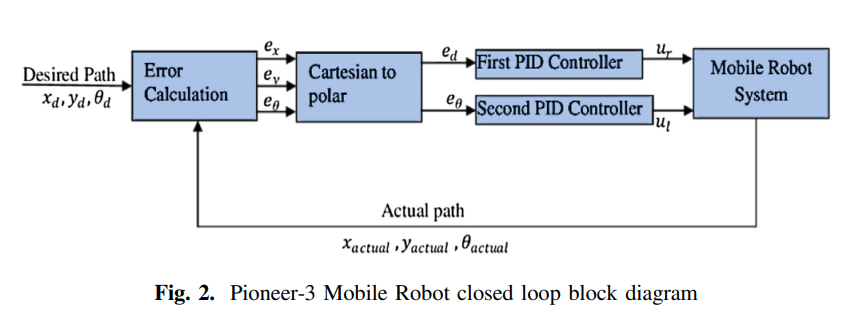Conference Paper

# Robust Path Tracking of Mobile Robot Using Fractional Order PID Controller

By
Ammar H.H.
Azar A.T.

This paper represents the control of the Pioneer-3 Mobile Robot as a complex non-linear system which provides an object for research nonlinear system kinematics and dynamics analysis. In this paper, the system modeling and simulation is divided into two main parts. The first part is the modeling and simulation using MATLAB and the second part is the whole mechanical design and its characteristics as a function of the motor speed and the torque depending on the system using Virtual Robot Environment Program (V-REP). The study uses Proportional–Integral–Derivative (PID) and Fractional Order PID (FOPID) controllers to obtain a robust controller for the system. The linear velocity loop controls the robot wheels speeds using the motor speed feedback signal from the encoder. The angular velocity control loop keeps the robot always in the accepted angle boundary using a six-degree of freedom gyroscope and accelerometer as a feedback signal. A state space model is obtained considering some assumptions and simplifications. This paper also studies and compares the results of two controllers PID and FOPID controllers from analysis perspectives with different optimization methods. The results demonstrated that the FOPID controller is superior in performance to the traditional PID controller. © 2020, Springer Nature Switzerland AG.